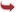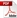International Journal of Scientific and Research Publications

#### IJSRP, Volume 6, Issue 6, June 2016 Edition [ISSN 2250-3153]Enhancement of Data Security on Transmission Network Using Fuzzy Logic
Onwughalu, M.K, Ogwata, C.M
Abstract: Security of data is an important factor in data transmission through network. This paper proposed a new method using fuzzy set theory to enhance the security. The data in the form of text to be transmitted is encrypted by using the AES Rijndael algorithm. The encryption algorithm is the mathematical procedure for performing encryption of data. A key is used to cipher a message and to decipher it back to the original message. Then the scrambled encrypted text is converted into numeric form by applying the fuzzy set theory. The fuzzy logic provides the text in the zero to one value. These numerical values before decryption are again converted into scrambled text. if the key provided by the user is the same key that is used for the encryption then original data will be retrieved. The paper, integrates the encryption of text and conversion of the unscrambled text from numerical to original by using fuzzy logic.
[VIEW FULL PAPER][DOWNLOAD]

Reference this Research Paper (copy & paste below code):

Onwughalu, M.K, Ogwata, C.M (2018); Enhancement of Data Security on Transmission Network Using Fuzzy Logic; Int J Sci Res Publ 6(6) (ISSN: 2250-3153). http://www.ijsrp.org/research-paper-0616.php?rp=P545483